### Introduction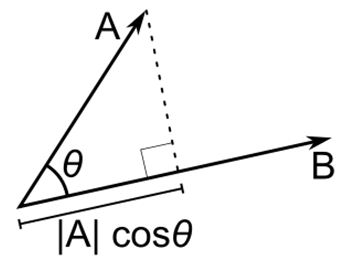If A and B are two vectors, the projection of A on B is the vector C with the same direction as B and with the length: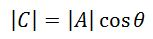When θ is not known, we can compute cos⁡θ using the following property of Dot Product A∙B: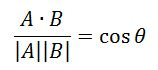Thus, the length of C can be also computed as follows: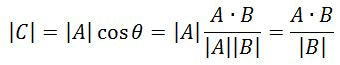Since C is in the same direction as B,Where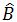is the Unit Vector with the same direction as B:### References & Resources

• Wikipedia - http://en.wikipedia.org/wiki/Vector_projection